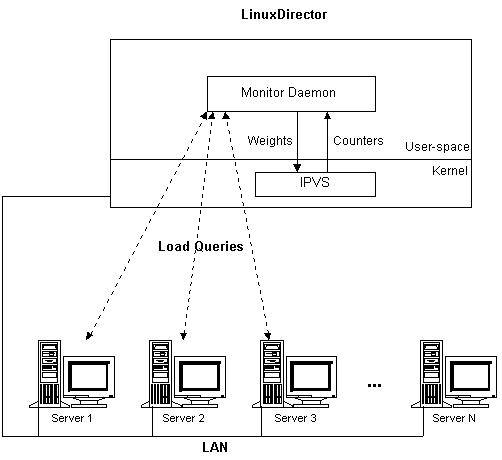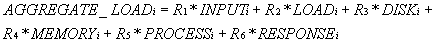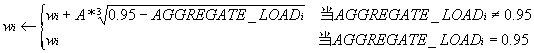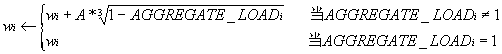# LVS集群的负载调度

1. 前言
LVS集群中实现的三种IP负载均衡技术，它们主要解决系统的可伸缩性和透明性问题，如何通过负载调度器将请求高 效地分发到不同的服务器执行，使得由多台独立计算机组成的集群系统成为一台虚拟服务器；客户端应用程序与集群系统交互时，就像与一台高性能的服务器交互一 样。

2. 内核中的连接调度算法

IPVS在内核中的负载均衡调度是以连接为粒度的。在HTTP协议（非持久）中，每个对象从WEB服务器上获取都需要建立一个TCP连接，同一用户 的不同请求会被调度到不同的服务器上，所以这种细粒度的调度在一定程度上可以避免单个用户访问的突发性引起服务器间的负载不平衡。

• 轮叫调度（Round-Robin Scheduling）
• 加权轮叫调度（Weighted Round-Robin Scheduling）
• 最小连接调度（Least-Connection Scheduling）
• 加权最小连接调度（Weighted Least-Connection Scheduling）
• 基于局部性的最少链接（Locality-Based Least Connections Scheduling）
• 带复制的基于局部性最少链接（Locality-Based Least Connections with Replication Scheduling）
• 目标地址散列调度（Destination Hashing Scheduling）
• 源地址散列调度（Source Hashing Scheduling）

2.1. 轮叫调度

 `````` 假设有一组服务器S = {S0, S1, …, Sn-1}，一个指示变量i表示上一次选择的 服务器，W(Si)表示服务器Si的权值。变量i被初始化为n-1，其中n > 0。 j = i; do { j = (j + 1) mod n; if (W(Sj) > 0) { i = j; return Si; } } while (j != i); return NULL; ``````

2.2. 加权轮叫调度

 `````` 假设有一组服务器S = {S0, S1, …, Sn-1}，W(Si)表示服务器Si的权值，一个 指示变量i表示上一次选择的服务器，指示变量cw表示当前调度的权值，max(S) 表示集合S中所有服务器的最大权值，gcd(S)表示集合S中所有服务器权值的最大 公约数。变量i初始化为-1，cw初始化为零。 while (true) { i = (i + 1) mod n; if (i == 0) { cw = cw - gcd(S); if (cw <= 0) { cw = max(S); if (cw == 0) return NULL; } } if (W(Si) >= cw) return Si; } ``````

2.3. 最小连接调度

 `````` 假设有一组服务器S = {S0, S1, ..., Sn-1}，W(Si)表示服务器Si的权值， C(Si)表示服务器Si的当前连接数。 for (m = 0; m < n; m++) { if (W(Sm) > 0) { for (i = m+1; i < n; i++) { if (W(Si) <= 0) continue; if (C(Si) < C(Sm)) m = i; } return Sm; } } return NULL; ``````

2.4. 加权最小连接调度

 `````` 假设有一组服务器S = {S0, S1, ..., Sn-1}，W(Si)表示服务器Si的权值， C(Si)表示服务器Si的当前连接数。所有服务器当前连接数的总和为 CSUM = ΣC(Si) (i=0, 1, .. , n-1)。当前的新连接请求会被发送服务器Sm， 当且仅当服务器Sm满足以下条件 (C(Sm) / CSUM)/ W(Sm) = min { (C(Si) / CSUM) / W(Si)} (i=0, 1, . , n-1) 其中W(Si)不为零 因为CSUM在这一轮查找中是个常数，所以判断条件可以简化为 C(Sm) / W(Sm) = min { C(Si) / W(Si)} (i=0, 1, . , n-1) 其中W(Si)不为零 因为除法所需的CPU周期比乘法多，且在Linux内核中不允许浮点除法，服务器的 权值都大于零，所以判断条件C(Sm) / W(Sm) > C(Si) / W(Si) 可以进一步优化 为C(Sm)*W(Si) > C(Si)* W(Sm)。同时保证服务器的权值为零时，服务器不被调 度。所以，算法只要执行以下流程。 for (m = 0; m < n; m++) { if (W(Sm) > 0) { for (i = m+1; i < n; i++) { if (C(Sm)*W(Si) > C(Si)*W(Sm)) m = i; } return Sm; } } return NULL; ``````

2.5. 基于局部性的最少链接调度

LBLC调度算法先根据请求的目标IP地址找出该目标IP地址最近使用的服务器，若该服务器是可用的且没有超载，将请求发送到该服务器；若服务器不 存在，或者该服务器超载且有服务器处于其一半的工作负载，则用“最少链接”的原则选出一个可用的服务器，将请求发送到该服务器。该算法的详细流程如下：

LBLC调度算法流程

 `````` 假设有一组服务器S = {S0, S1, ..., Sn-1}，W(Si)表示服务器Si的权值， C(Si)表示服务器Si的当前连接数。ServerNode[dest_ip]是一个关联变量，表示 目标IP地址所对应的服务器结点，一般来说它是通过Hash表实现的。WLC(S)表示 在集合S中的加权最小连接服务器，即前面的加权最小连接调度。Now为当前系统 时间。 if (ServerNode[dest_ip] is NULL) then { n = WLC(S); if (n is NULL) then return NULL; ServerNode[dest_ip].server = n; } else { n = ServerNode[dest_ip].server; if ((n is dead) OR (C(n) > W(n) AND there is a node m with C(m) < W(m)/2))) then { n = WLC(S); if (n is NULL) then return NULL; ServerNode[dest_ip].server = n; } } ServerNode[dest_ip].lastuse = Now; return n; ``````

2.6. 带复制的基于局部性最少链接调度

LBLCR算法先根据请求的目标IP地址找出该目标IP地址对应的服务器组；按“最小连接”原则从该服务器组中选出一台服务器，若服务器没有超载， 将请求发送到该服务器；若服务器超载；则按“最小连接”原则从整个集群中选出一台服务器，将该服务器加入到服务器组中，将请求发送到该服务器。同时，当该 服务器组有一段时间没有被修改，将最忙的服务器从服务器组中删除，以降低复制的程度。LBLCR调度算法的流程如下：

LBLCR调度算法流程

 `````` 假设有一组服务器S = {S0, S1, ..., Sn-1}，W(Si)表示服务器Si的权值， C(Si)表示服务器Si的当前连接数。ServerSet[dest_ip]是一个关联变量，表示 目标IP地址所对应的服务器集合，一般来说它是通过Hash表实现的。WLC(S)表示 在集合S中的加权最小连接服务器，即前面的加权最小连接调度；WGC(S)表示在 集合S中的加权最大连接服务器。Now为当前系统时间，lastmod表示集合的最近 修改时间，T为对集合进行调整的设定时间。 if (ServerSet[dest_ip] is NULL) then { n = WLC(S); if (n is NULL) then return NULL; add n into ServerSet[dest_ip]; } else { n = WLC(ServerSet[dest_ip]); if ((n is NULL) OR (n is dead) OR (C(n) > W(n) AND there is a node m with C(m) < W(m)/2))) then { n = WLC(S); if (n is NULL) then return NULL; add n into ServerSet[dest_ip]; } else if (|ServerSet[dest_ip]| > 1 AND Now - ServerSet[dest_ip].lastmod > T) then { m = WGC(ServerSet[dest_ip]); remove m from ServerSet[dest_ip]; } } ServerSet[dest_ip].lastuse = Now; if (ServerSet[dest_ip] changed) then ServerSet[dest_ip].lastmod = Now; return n; ``````

2.7. 目标地址散列调度

 `````` 假设有一组服务器S = {S0, S1, ..., Sn-1}，W(Si)表示服务器Si的权值， C(Si)表示服务器Si的当前连接数。ServerNode[]是一个有256个桶（Bucket）的 Hash表，一般来说服务器的数目会运小于256，当然表的大小也是可以调整的。 算法的初始化是将所有服务器顺序、循环地放置到ServerNode表中。若服务器的 连接数目大于2倍的权值，则表示服务器已超载。 n = ServerNode[hashkey(dest_ip)]; if ((n is dead) OR (W(n) == 0) OR (C(n) > 2*W(n))) then return NULL; return n; ``````

 `````` static inline unsigned hashkey(unsigned int dest_ip) { return (dest_ip* 2654435761UL) & HASH_TAB_MASK; } 其中，2654435761UL是2到2^32 (4294967296)间接近于黄金分割的素数， (sqrt(5) - 1) / 2 = 0.618033989 2654435761 / 4294967296 = 0.618033987 ``````

2.8. 源地址散列调度

3. 动态反馈负载均衡算法3.1. 连接调度

3.1.1. 简单连接调度

3.1.2. 实际TCP/IP流量的特征

3.2. 动态反馈负载均衡机制

TCP/IP流量的特征通俗地说是有许多短事务和一些长事务组成，而长事务的工作量在整个工作量占有较高的比例。所以，我们要设计一种负载均衡算法，来避免长事务的请求总被分配到一些机器上，而是尽可能将带有毛刺（Burst）的分布分割成相对较均匀的分布。

3.3. 综合负载3.4. 权值计算3.5. 一个实现例子4. 小结

• 轮叫调度（Round-Robin Scheduling）
• 加权轮叫调度（Weighted Round-Robin Scheduling）
• 最小连接调度（Least-Connection Scheduling）
• 加权最小连接调度（Weighted Least-Connection Scheduling）
• 基于局部性的最少链接（Locality-Based Least Connections Scheduling）
• 带复制的基于局部性最少链接（Locality-Based Least Connections with Replication Scheduling）
• 目标地址散列调度（Destination Hashing Scheduling）
• 源地址散列调度（Source Hashing Scheduling）